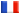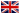# Séminaire de Cryptographie

## Xavier Pujol### Analysis of BKZ

Strong lattice reduction is the key element for most attacks against lattice-based cryptosystems. Between the strongest but impractical HKZ reduction and the weak but fast LLL reduction, there have been several attempts to find efficient trade-offs. Among them, the BKZ algorithm introduced by Schnorr and Euchner in 1991 seems to achieve the best time/quality compromise in practice. However, no reasonable time complexity upper bound was known so far for BKZ. We give a proof that after O~(n^3/k^2) calls to a k-dimensional HKZ reduction subroutine, BKZ_k returns a basis such that the norm of the first vector is at most ~= gamma_k ^ (n/2(k-1)) * det(L)^(1/n). The main ingredient of the proof is the analysis of a linear dynamic system related to the algorithm.